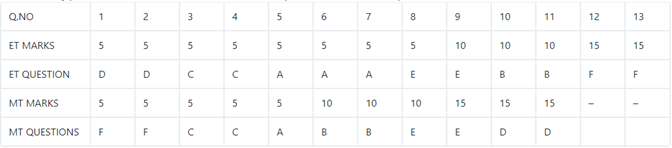### CAT 2019 - Slot 1 - Logical Reasoning and Data Interpretation - The first year students in a business school are split into six sections

\$ 39
99
Monthly
• List Item #1
• List Item #2
Popular
##### The first year students in a business school are split into six sections. In 2019 the Business Statistics course was taught in these six sections by Annie, Beti, Chetan, Dave, Esha, and Fakir. All six sections had a common midterm (MT) and a common endterm (ET) worth 100 marks each. ET contained more questions than MT. Questions for MT and ET were prepared collectively by the six faculty members. Considering MT and ET together, each faculty member prepared the same number of questions. Each of MT and ET had at least four questions that were worth 5 marks, at least three questions that were worth 10 marks, and at least two questions that were worth 15 marks. In both MT and ET, all the 5-mark questions preceded the 10-mark questions, and all the 15- mark questions followed the 10-mark questions. The following additional facts are known. i. Annie prepared the fifth question for both MT and ET. For MT, this question carried 5 marks. ii. Annie prepared one question for MT. Every other faculty member prepared more than one questions for MT. iii. All questions prepared by a faculty member appeared consecutively in MT as well as ET. iv. Chetan prepared the third question in both MT and ET; and Esha prepared the eighth question in both. v. Fakir prepared the first question of MT and the last one in ET. Dave prepared the last question of MT and the first one in ET.

Q. 1: The second question in ET was prepared by:
1. Chetan
2. Esha
3. Beti
4. Dave

##### Q. 2: How many 5- mark questions were there in MT and ET combined?1. 132. 103. 124. Cannot be determined

Q. 3: Who prepared 15-mark questions for MT and ET?
1. Only Dave, Esha and Fakir
2. Only Beti, Dave, Esha and Fakir
3. Only Esha and Fakir
4. Only Dave and Fakir

Q. 4: Which of the following questions did Beti prepare in ET?
1. Tenth question
2. Ninth question
3. Fourth question
4. Seventh question

1. Option 4

2. Option  1

3. Option  1

4. Option 1

As both MT and ET has atleast 4 questions of 5 marks, atleast 3 ques of 10 marks, and 2 questions of 15 marks

Marks covered by these = 5×4 + 10×3 + 15×2 = 80

Marks left = 20

20 can be divided as (5,5,5,5) (5,5,10) (5,15) (10,10)

Order of question = 5 marks, 10 marks, 15 marks

Since it is given that 5th question is a 5 mark question case of 10,10 marks is rued out

As everyone prepared an equa number of questions the number has to be a factor of 6 greater than 18 and the only possible number here is 24.

Hence the only possible cases are (5,5,5,5) (5,15)Thus ET has 13 questions and MT has 11 questionsNow all the question can be answered.

Inspiring Education… Assuring Success!!

Ghatkopar | Borivali | Andheri | Online